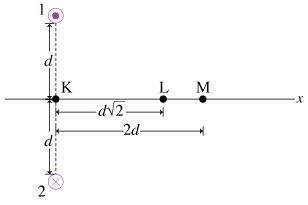# Problem: Part APoint M is located a distance 2d from the midpoint between the two wires. Find the magnitude of the magnetic field B1M created at point M by wire 1.Express your answer in terms of I, d, and appropriate constants.Part BFind the magnitude of the net magnetic field BM created at point M by both wires.Express your answer in terms of I, d, and appropriate constants.

###### FREE Expert Solution

This problem involves the magnetic field around a straight current-carrying conductor.

Magnetic field around a wire carrying current:

$\overline{){\mathbf{B}}{\mathbf{=}}\frac{{\mathbf{\mu }}_{\mathbf{0}}\mathbf{i}}{\mathbf{2}\mathbf{\pi }\mathbf{r}}}$

89% (380 ratings)###### Problem DetailsPart A

Point M is located a distance 2d from the midpoint between the two wires. Find the magnitude of the magnetic field B1M created at point M by wire 1.

Part B

Find the magnitude of the net magnetic field BM created at point M by both wires.